Note: The other languages of the website are Google-translated. Back to English

## 如何在Excel中拼出數字或將數字轉換為英語單詞？

#### 用VBA代碼拼出數字或將數字轉換為英文單詞

1。 按住 ALT + F11 鍵，然後打開 Microsoft Visual Basic for Applications 窗口。

2。 點擊 插入 > 模塊，然後將以下宏粘貼到 模塊窗口。

VBA：在Excel中將貨幣數字拼寫為英文單詞

``````Function SpellNumberToEnglish(ByVal pNumber)
'Updateby20131113
Dim Dollars, Cents
arr = Array("", "", " Thousand ", " Million ", " Billion ", " Trillion ")
pNumber = Trim(Str(pNumber))
xDecimal = InStr(pNumber, ".")
If xDecimal > 0 Then
Cents = GetTens(Left(Mid(pNumber, xDecimal + 1) & "00", 2))
pNumber = Trim(Left(pNumber, xDecimal - 1))
End If
xIndex = 1
Do While pNumber <> ""
xHundred = ""
xValue = Right(pNumber, 3)
If Val(xValue) <> 0 Then
xValue = Right("000" & xValue, 3)
If Mid(xValue, 1, 1) <> "0" Then
xHundred = GetDigit(Mid(xValue, 1, 1)) & " Hundred "
End If
If Mid(xValue, 2, 1) <> "0" Then
xHundred = xHundred & GetTens(Mid(xValue, 2))
Else
xHundred = xHundred & GetDigit(Mid(xValue, 3))
End If
End If
If xHundred <> "" Then
Dollars = xHundred & arr(xIndex) & Dollars
End If
If Len(pNumber) > 3 Then
pNumber = Left(pNumber, Len(pNumber) - 3)
Else
pNumber = ""
End If
xIndex = xIndex + 1
Loop
Select Case Dollars
Case ""
Dollars = "No Dollars"
Case "One"
Dollars = "One Dollar"
Case Else
Dollars = Dollars & " Dollars"
End Select
Select Case Cents
Case ""
Cents = " and No Cents"
Case "One"
Cents = " and One Cent"
Case Else
Cents = " and " & Cents & " Cents"
End Select
SpellNumberToEnglish = Dollars & Cents
End Function
Function GetTens(pTens)
Dim Result As String
Result = ""
If Val(Left(pTens, 1)) = 1 Then
Select Case Val(pTens)
Case 10: Result = "Ten"
Case 11: Result = "Eleven"
Case 12: Result = "Twelve"
Case 13: Result = "Thirteen"
Case 14: Result = "Fourteen"
Case 15: Result = "Fifteen"
Case 16: Result = "Sixteen"
Case 17: Result = "Seventeen"
Case 18: Result = "Eighteen"
Case 19: Result = "Nineteen"
Case Else
End Select
Else
Select Case Val(Left(pTens, 1))
Case 2: Result = "Twenty "
Case 3: Result = "Thirty "
Case 4: Result = "Forty "
Case 5: Result = "Fifty "
Case 6: Result = "Sixty "
Case 7: Result = "Seventy "
Case 8: Result = "Eighty "
Case 9: Result = "Ninety "
Case Else
End Select
Result = Result & GetDigit(Right(pTens, 1))
End If
GetTens = Result
End Function
Function GetDigit(pDigit)
Select Case Val(pDigit)
Case 1: GetDigit = "One"
Case 2: GetDigit = "Two"
Case 3: GetDigit = "Three"
Case 4: GetDigit = "Four"
Case 5: GetDigit = "Five"
Case 6: GetDigit = "Six"
Case 7: GetDigit = "Seven"
Case 8: GetDigit = "Eight"
Case 9: GetDigit = "Nine"
Case Else: GetDigit = ""
End Select
End Function
``````

3。 然後保存代碼，並返回工作表，在相鄰的單元格B2中，輸入公式 = SpellNumberToEnglish（A1），請參見屏幕截圖：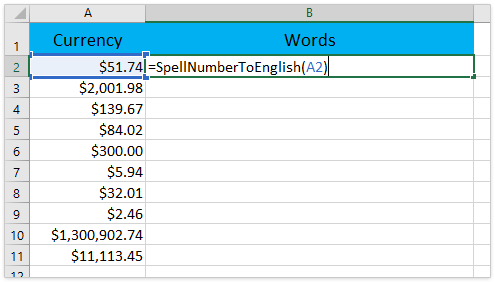4。 然後按 Enter 鍵，然後選擇單元格B2，然後根據需要將填充手柄拖到包含此公式的範圍內。 並且所有貨幣編號均已拼寫為英文單詞。 看截圖：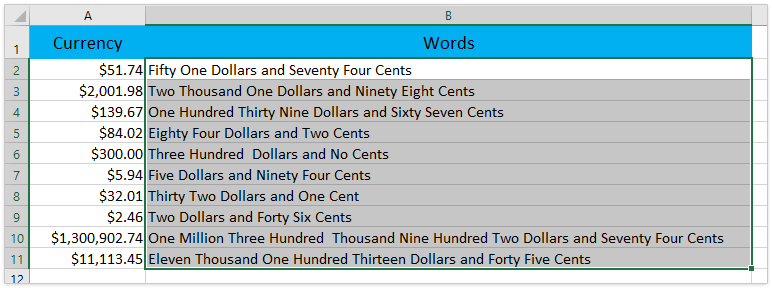#### 使用Kutools for Excel拼出數字或將數字轉換為英文單詞

Excel的Kutools- 包括 300 多個方便的 Excel 工具。 全功能免費試用 30-天，無需信用卡！ 立即行動吧!

1。 選擇要拼寫的範圍號，然後單擊 庫工具 > 內容 > 數字到單詞.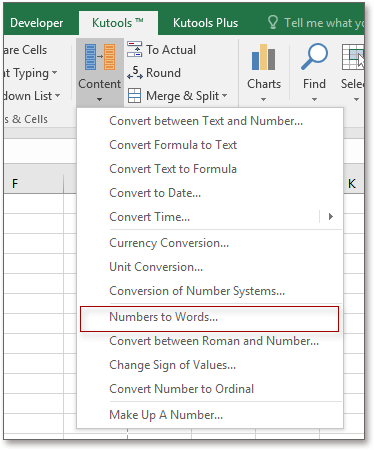2。 在彈出的對話框中，選擇 English 來自 語言。 您可以從右側預覽結果 產品規格書預覽 窗格。 看截圖：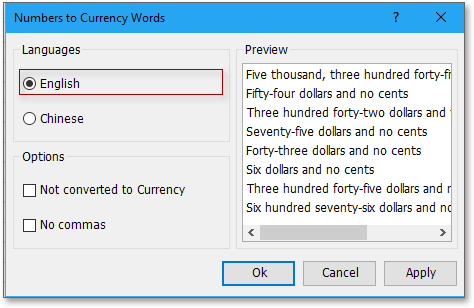3。 然後點擊 Ok or 登記。 並且所有價格金額數字都已轉換為原始範圍內的文本字符串。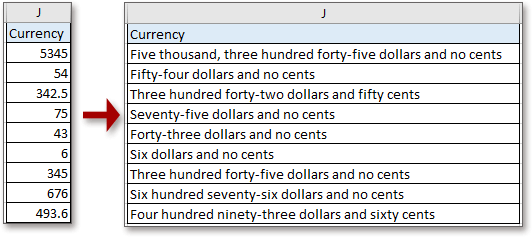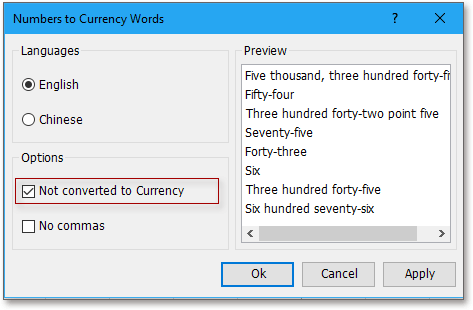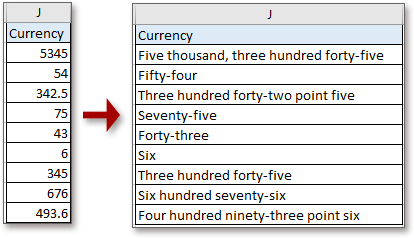### 最佳辦公效率工具

#### Kutools for Excel 解決了你的大部分問題，並將你的生產力提高了 80%

• 重用: 快速插入 複雜的公式，圖表 以及您以前使用過的任何東西； 加密單元 帶密碼 創建郵件列表 並發送電子郵件...
• 超級公式欄 （輕鬆編輯多行文本和公式）； 閱讀版式 （輕鬆讀取和編輯大量單元格）； 粘貼到過濾範圍...
• 合併單元格/行/列 不會丟失數據； 拆分單元格內容； 合併重複的行/列...防止細胞重複； 比較範圍...
• 選擇重複或唯一 行; 選擇空白行 （所有單元格都是空的）； 超級查找和模糊查找 在許多工作簿中； 隨機選擇...
• 確切的副本 多個單元格，無需更改公式參考； 自動創建參考 到多張紙； 插入項目符號，複選框等...
• 提取文字，添加文本，按位置刪除， 刪除空間; 創建和打印分頁小計； 在單元格內容和註釋之間轉換...
• 超級濾鏡 （將過濾方案保存並應用於其他工作表）； 高級排序 按月/週/日，頻率及更多； 特殊過濾器 用粗體，斜體...
• 結合工作簿和工作表; 根據關鍵列合併表； 將數據分割成多個工作表; 批量轉換xls，xlsx和PDF...
• 超過 300 項強大的功能. 支持 Office / Excel 2007-2021 和 365。支持所有語言。 在您的企業或組織中輕鬆部署。 完整功能 30 天免費試用。 60 天退款保證。#### Office選項卡為Office帶來了選項卡式界面，使您的工作更加輕鬆

• 在Word，Excel，PowerPoint中啟用選項卡式編輯和閱讀，發布者，Access，Visio和Project。
• 在同一窗口的新選項卡中而不是在新窗口中打開並創建多個文檔。
• 將您的工作效率提高 50%，每天為您減少數百次鼠標點擊！plx 讓我清楚地知道，我怎樣才能以我所在國家/地區的貨幣格式查看它，即盧比代替美元，派薩代替美分。

THNX 彌補了這些提示

0   字符

## 關注我們

Microsoft和Office徽標是Microsoft Corporation在美國和/或其他國家的商標或註冊商標。